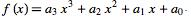# Test: Introduction To Polynomials

## 20 Questions MCQ Test Mathematics (Maths) Class 9 | Test: Introduction To Polynomials

Description
This mock test of Test: Introduction To Polynomials for Class 9 helps you for every Class 9 entrance exam. This contains 20 Multiple Choice Questions for Class 9 Test: Introduction To Polynomials (mcq) to study with solutions a complete question bank. The solved questions answers in this Test: Introduction To Polynomials quiz give you a good mix of easy questions and tough questions. Class 9 students definitely take this Test: Introduction To Polynomials exercise for a better result in the exam. You can find other Test: Introduction To Polynomials extra questions, long questions & short questions for Class 9 on EduRev as well by searching above.
QUESTION: 1

Solution:
QUESTION: 2

Solution:
QUESTION: 3

### Solution of a quadratic equation x²+ 5x - 6 = 0

Solution:

x²+ 5x - 6 = 0
x² - x + 6x - 6
x (x-1) + 6 (x-1)
(x-1) (x+6)
x = 1; x = -6

QUESTION: 4

If 3 + 5 – 8 = 0, then the value of (3)3 + (5)3 – (8)3 is

Solution:

3^3+5^3-8^3=27+125-512

=152-512

=-360

QUESTION: 5

Solution of a quadratic equation x²+ 5x - 6 = 0

Solution:

⇒ x²+ 5x - 6 = 0

⇒ x²+ 6x -x - 6 = 0

⇒ x(x+6) -1(x+6) = 0

⇒ (x-1) (x+6) = 0

⇒ x = 1, -6

QUESTION: 6

If x + 2 is a factor of x3 – 2ax2 + 16, then value of a is

Solution:

use factor theorem as x+2 is factor of

x³-2ax²+16 so put x = -2 and equate the equation to 0

so putting x = -2

(-2)³-2a(-2)²+16 =0

-8-8a+16=0

-8a = -8

a = 8/8= 1

So,

a = 1

QUESTION: 7

Which of the following is not a quadratic polynomial?​

Solution:
QUESTION: 8

P of x = ax, a is not equal to 0. find zeros of polynmial

Solution:

p(x) = ax
p(x) = 0
ax = 0
x = 0/a
x = 0
{0 by something is equals to 0}
Therefore,0 is the zero of the polynomial.
Checking:-
p(0) = a(0)
= 0

QUESTION: 9

Zero of the polynomial p(x) where p (x) = ax, a ≠ 0 is:

Solution:
QUESTION: 10

If x + 2 is a factor of x3 – 2ax2 + 16, then value of a is

Solution:
QUESTION: 11

If p(x) = 7 – 3x + 2x2 then value of p(-2) is:

Solution:

p(-2) = 7 - 3(-2) + 2(-2)2

= 7+6+8 = 21

QUESTION: 12

A linear polynomials has how many zeros

Solution:

A linear polynomial has 1 zero.

A quadratic polynomial has 2 zeroes.

A cubic polynomial has 3 zeroes.

In general, any polynomial has as many zeroes as its degree.

QUESTION: 13

If one of the factor of x2 + x – 20 is (x + 5). Find the other

Solution:
QUESTION: 14

Which of the following is a quadratic polynomial in one variable?

Solution:
QUESTION: 15

A cubic polynomial is a polynomial of degree …………

Solution:

A cubic polynomial is a polynomial of degree 3. A univariate cubic polynomial has the formAn equation involving a cubic polynomial is called a cubic equation. A closed-form solution known as the cubic formula exists for the solutions of an arbitrary cubic equation.

QUESTION: 16

Find the value of the polynomial 6 – 4x + 3x2 at x = 3

Solution:
QUESTION: 17

Degree of zero polynomial is:

Solution:

A zero polynomial is a polynomial in which all the coefficients are 0.

Let the polynomials highest power variable be x^n as per the above statement x^n = 0

Now, The degree of the zero polynomial is log0 which is undefined.

Hence. The degree of zero polynomial is undefined.

QUESTION: 18

Zero of a zero polynomial is:

Solution:

Zero of the zero polynomial is any real number.
e.g., Let us consider zero polynomial be 0(x-k), where k is a real number For determining the zero, put x-k = 0 ⇒ x = k Hence, zero of the zero polynomial be any real number.

QUESTION: 19

What is the coefficient of x in  x3 + 3x2 - 2x - 1

Solution:
QUESTION: 20

The degree of the polynomial x4 – 3x3 + 2x2 – 5x + 3 is:

Solution:

The degree refers to the highest power of the polynomial. In this polynomial X has highest power 4.So the degree of polynomial is 4.